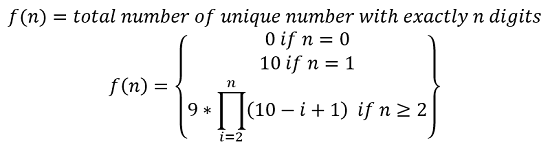Q:

# Count Numbers with unique digits

Given a non-negative integer n, count all numbers with unique digits, x0<=x<10^n.

```    Input & output:
n=2
Total numbers that can be formed is 91
```

Explanation with example

```    For n =2.

Total numbers = 0 to 102 (excluding)
except 11, 22, 33, 44, 55, 66, 77, 88, 99 which has repeated digits.```

• If number of digits is 0, then unique numbers that can be formed is 0
• If number of digits is 1, then unique numbers that can be formed is 10
0, 1, 2, 3, 4, 5, 6, 7, 8, 9
• If number of digits is 2,
Then for the first digit (from left), we have 9 choices (excluding 0) [1 to 9]
And for second digit we have 9 choices (0 to 10 except the previous digit)
So basically, the number is:
ij where i=[1,9] and j=[0,9]and i≠j
So, total number possible are 9*9=81
• If number of digits is 3,
Then for first digit (from left), we have 9 choices (excluding 0) [1 to 9]
And for second digit we have 9 choices (0 to 10 except the previous digit)
And for third digit we have 8 choices (0 to 10 except the previous two digits)
So basically, the number is:
ijk where i=[1,9] and j,k=[0,9]and i≠j & j≠k,i
So, total number possible are 9*9*8=648
So, if number of digits is i, then the ith digit will have (10-i+1) choices
So, we can formulateNow this above recursion can be implemented using dynamic programming.

```    DP[i] = f(i)=total number of unique number with exactly i digits
```

```    1)  Initialize DP[n+1]  with 0
2)  DP=0,DP=10,DP=81
3)  Result = count of total numbers(x) that can be
formed with unique digits,where 0<=x<10^n
4)  Base value of result=91(for n=2)
5)  for i=3 to n
DP[i]=DP[i-1]*(10-i+1);
result=result+DP[i];
end for
6)  Return result
```

C++ Implementation:

``````#include <bits/stdc++.h>
using namespace std;

int countNumbersWithUniqueDigits(int n)
{
//base cases
if (n == 0)
return 0;
if (n == 1)
return 10;
if (n == 2)
return 91;

//base value
long long int DP[n + 1];
DP = 0;
DP = 10;
DP = 81;
long long int res = 91;

for (int i = 3; i <= n; i++) {
//compute f(i)
DP[i] = DP[i - 1] * (10 - i + 1);
}
//result
return res;
}

int main()
{
int n;

cout << "Enter the number\n";
cin >> n;

int ans = countNumbersWithUniqueDigits(n);
cout << "Total number count is: " << ans << endl;

return 0;
}
``````

Output

```RUN 1:
Enter the number
3
Total number count is: 739

RUN 2:
Enter the number
5
Total number count is: 32491```